• Bresenham，DDA,中点分割算法直线绘制，并且有效率对比，还有圆弧以及圆的绘制算法Bresenham
• 计算机图形学—DDA直线画法c#实现 DDA直线画法，即数值微分法，是计算机图形学中直线段扫描转换的经典算法之一，本篇文章将简单介绍DDA...运用这思想，算出每一步y的取值，并对y舍五入得到像素值，即可得到直线段每

计算机图形学—DDA直线画法c#实现

DDA直线画法，即数值微分法，是计算机图形学中直线段扫描转换的经典算法之一，本篇文章将简单介绍DDA算法，并给出c#实现的代码。

一、DDA直线画法

设直线的斜截式方程为 y i = k i x + b y_i = k_ix + b
根据微分的思想，可以得到 y i + 1 = y i + k y_{i+1} = y_i + k
这个式子的含义是：当前步的y值等于前一步的y值加上斜率。运用这个思想，算出每一步y的取值，并对y四舍五入得到像素值，即可得到直线段每个像素点的坐标并绘制。
下面是一个简单的举例：绘制直线段（0，0）–（5，2）。

xy四舍五入
000
10+0.40
20.4+0.41
30.8+0.41
41.2+0.42
51.6+0.42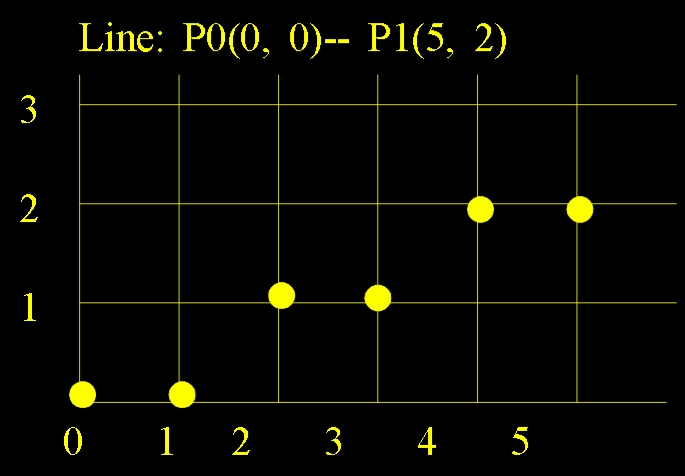以上例子仅讨论了k<=1的情况，k>1的情况可同理推出，在代码实现中有讨论，不再举例。

二、C#代码实现

像素点的绘制采用System.Drawing库中Graphics的FillRectangle方法填充单个像素。

public void DDAline(int x0, int y0, int x1, int y1, Graphics g, Brush brush) //DDA画线法。
{
float dx, dy, k;
dx = x1 - x0;
dy = y1 - y0;
if (dx == 0)//斜率不存在情况
{
int y;
for (y = y0; y <= y1; y++)
{
g.FillRectangle(brush, x0, y, 1, 1);
}
for (y = y1; y <= y0; y++)
{
g.FillRectangle(brush, x0, y, 1, 1);
}
}
else
{
k = dy / dx;
if (Math.Abs(k) <= 1)//斜率小于1情况。
{
int x;
float y = y0;
for (x = x0; x <= x1; x++)//x0小于x1情况。
{
g.FillRectangle(brush, x, (int)Math.Floor(y + 0.5), 1, 1);
y += k;
}
y = y1;
for (x = x1; x <= x0; x++)//x1小于x0情况。
{
g.FillRectangle(brush, x, (int)Math.Floor(y + 0.5), 1, 1);
y += k;
}
}
if (Math.Abs(k) > 1)//斜率大于1情况。
{
float x = x0;
int y;
for (y = y0; y <= y1; y++)//y0小于y1情况。
{
g.FillRectangle(brush, (int)Math.Floor(x + 0.5), y, 1, 1);
x += 1 / k;
}
x = x1;
for (y = y1; y <= y0; y++)//y1小于y0情况。
{
g.FillRectangle(brush, (int)Math.Floor(x + 0.5), y, 1, 1);
x += 1 / k;
}
}
}
}

总结

代码实现过程中，应考虑直线画法的多种情况，避免代码错误。例如考虑斜率不存在的情况，起点x0与终点x1的大小关系。

展开全文c# 计算机视觉
• #include <GL/glut.h>...void dda_line(int x1, int y1, int x2, int y2) { glPointSize(1.0); glBegin(GL_POINTS); glVertex2i(x1, y1); glVertex2i(x2, y2); // x,y的增量 int dx = x
#include <GL/glut.h>
#include <stdio.h>
#include <cstdlib>
#include <windows.h>
void dda_line(int x1, int y1, int x2, int y2) {
glPointSize(1.0);
glBegin(GL_POINTS);
glVertex2i(x1, y1);
glVertex2i(x2, y2);
// x,y的增量
int dx = x2 - x1;
int dy = y2 - y1;
double dert_x;
double dert_y;
double x, y;
int steps;
// 步长，看x和y哪个大
if (abs(dx) > abs(dy))
steps = abs(dx);
else
steps = abs(dy);
// 根据步长，算x和y的增量
dert_x = (GLdouble)dx / (GLdouble)steps;
dert_y = (GLdouble)dy / (GLdouble)steps;
x = x1;
y = y1;
for (int i = 1; i <= steps; i++) {
x += dert_x;
y += dert_y;
glVertex2f(x, y);
}
glEnd();
}

void myDisplay(void) {
int x0 = 250;
int y0 = 250;
int x1 = 500;
int y1 = 250;
while (true)
{
glClear(GL_COLOR_BUFFER_BIT);
dda_line(x0, y0, x1, y1);
if (x1 > 250 && y1 <= 250) {
x1 -= 1;
y1 -= 1;
}
else if (x1 <= 250 && y1 < 250)
{
x1 -= 1;
y1 += 1;
}
else if (x1 < 250 && y1 >= 250)
{
x1 += 1;
y1 += 1;
}
else if (x1 >= 250 && y1 > 250)
{
x1 += 1;
y1 -= 1;
}
else
printf("error!!!, %d, %d", x1, y1);

Sleep(10);
glFlush();
}
glClear(GL_COLOR_BUFFER_BIT);
glFlush();
}
int main(int argc, char* argv[])
{
glutInit(&argc, argv);
glutInitDisplayMode(GLUT_RGB | GLUT_SINGLE);
glutInitWindowPosition(500, 200);
glutInitWindowSize(500, 500);
glutCreateWindow("DDA");
gluOrtho2D(0.0, 500.0, 0.0, 500.0);
glutDisplayFunc(&myDisplay);
glutMainLoop();
return 0;
}

展开全文算法
• DDA算法主要是利用了增量的思想，通过同时对x和y各增加一小增量，计算下一步的x和y值。 根据上式可知△x=1时，x每递增1，y就递增k，所以只需要对x和y不断递增就可以得到下一点的函数值，这样避免了对每一像素都...

一、直线算法

1、数值微分法（DDA算法）

DDA算法主要是利用了增量的思想，通过同时对x和y各增加一个小增量，计算下一步的x和y值。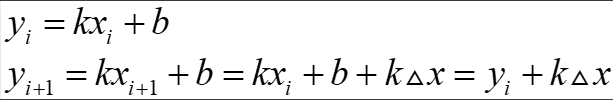根据上式可知△x=1时，x每递增1，y就递增k，所以只需要对x和y不断递增就可以得到下一点的函数值，这样避免了对每一个像素都使用直线方程来计算，消除了浮点数乘法运算。

#include <Windows.h>
#include <iostream>
#include <cmath>
using namespace std;

const int ScreenWidth = 500;
const int ScreenHeight = 500;

LRESULT CALLBACK WinProc(HWND hWnd, UINT message, WPARAM wParam, LPARAM lParam)
{
switch (message) {
case WM_CLOSE:
DestroyWindow(hWnd);
break;
case WM_DESTROY:
PostQuitMessage(0);
break;
default:
return DefWindowProc(hWnd, message, wParam, lParam);
break;
}
return 0;
}

void DDALine(int x0,int y0,int x1,int y1,HDC hdc)
{
int i=1;
float dx, dy, length, x, y;
if (fabs(x1 - x0) >= fabs(y1 - y0))
length = fabs(x1 - x0);
else
length = fabs(y1 - y0);
dx = (x1 - x0) / length;
dy = (y1 - y0) / length;
x = x0;
y = y0;
while (i<=length)
{
SetPixel(hdc,int(x + 0.5), ScreenHeight-40-int(y + 0.5), RGB(0, 0, 0));
x = x + dx;
y = y + dy;
i++;
}
}
int WINAPI WinMain(HINSTANCE hInstance, HINSTANCE hPrevInstance, PSTR szCmdLine, int nShowCmd)
{
WNDCLASS wcs;
wcs.cbClsExtra = 0;                                         // 窗口类附加参数
wcs.cbWndExtra = 0;                                         // 窗口附加参数
wcs.hbrBackground = (HBRUSH)GetStockObject(WHITE_BRUSH);    // 窗口DC背景
wcs.hCursor = LoadCursor(hInstance, IDC_CROSS);             // 鼠标样式
wcs.hIcon = LoadIcon(NULL, IDI_WINLOGO);                    // 窗口icon
wcs.hInstance = hInstance;                                  // 应用程序实例
wcs.lpfnWndProc = (WNDPROC)WinProc;
wcs.lpszClassName = "CG";
wcs.style = CS_VREDRAW | CS_HREDRAW;
RegisterClass(&wcs);
HWND hWnd;
hWnd = CreateWindow("CG","DrawLine", WS_OVERLAPPEDWINDOW, 200, 200, ScreenWidth, ScreenHeight, NULL, NULL, hInstance, NULL);
ShowWindow(hWnd, nShowCmd);
UpdateWindow(hWnd);
MSG msg;

// hdc init
HDC hdc = GetDC(hWnd);

// 绘图，画一条从点（0,0）到（100,350）的直线
DDALine(0, 0, 100, 350, hdc);// 消息循环
while (GetMessage(&msg, 0, NULL, NULL)) {
TranslateMessage(&msg);
DispatchMessage(&msg);
}

// release
ReleaseDC(hWnd, hdc);
return 0;
}

以上是DDA算法的实现，WinMain和WinProc函数是Windows API编程特有的，我们只需要关注DDALine这个绘图函数，该函数传入两个点的坐标画出一条直线。

首先判断起点和终点间x轴和y轴哪个轴向的跨度更大（斜率范围），为了防止丢失像素，应让跨度更大的轴向每次自增1，这样能获得更精确的结果。

接下来就没什么好说的，依次让x和y加上增量然后四舍五入就行了，浮点数四舍五入可以直接用int(x+0.5)计算，setPixel函数用于设置像素的颜色值（需要传入窗口的hdc句柄），由于Windows窗口坐标的原点在左上角，所以拿窗口高度减去y值就可以转换成平常习惯的左下角坐标系了。

2、Bresenham算法

DDA算法尽管消除了浮点数乘法运算，但仍存在浮点数加法和取整操作，效率仍有待提高，1965年Bresenham提出了更好的直线生成算法，成为了时至今日图形学领域使用最广泛的直线生成算法，该算法采用增量计算，借助一个误差量的符号确定下一个像素点的位置，该算法中不存在浮点数，只有整数运算，大大提高了运行效率。

我们先只考虑斜率在0-1之间的情况，从线段左端点开始处理，并逐步处理每个后续列，每确定当前列的像素坐标(xi,yi)后，那么下一步需要在列xi+1上确定y的值，此时y值要么不变，要么增加1，这是因为斜率在0-1之间，x增长比y快，所以x每增加1，y的增量是小于1的。

对于左端点默认为其像素坐标，下一列要么是右方的像素，要么是右上方的像素，设右上方像素到直线的距离为d2，右方像素到直线的距离为d1，显然只需要判断直线离哪个像素点更近也就是d1-d2的符号即可找到最佳像素。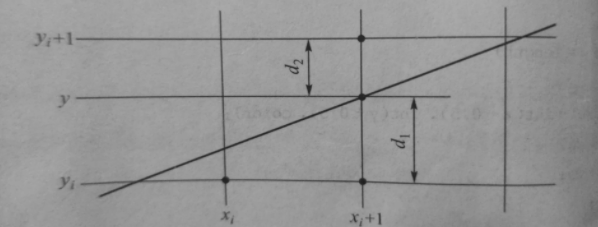所以可以推出以下式子：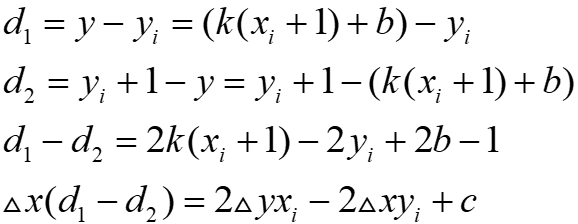其中△x起点到终点x轴上距离，△y为y轴上距离，k=△y/△x，c是常量，与像素位置无关。

令ei=△x(d1-d2),则ei的计算仅包括整数运算，符号与d1-d2一致，称为误差量参数，当它小于0时，直线更接近右方像素，大于0时直线更接近右上方像素。

可利用递增整数运算得到后继误差量参数，计算如下：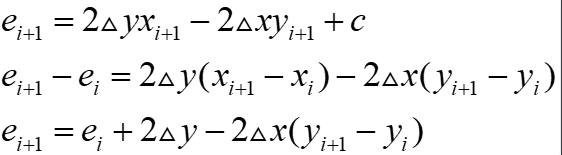所以选择右上方像素时(yi+1-yi=1)：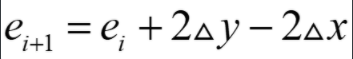选择右方像素时(yi+1-yi=0)：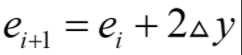初始时，将k=△y/△x代入△x(d1-d2)中可得到起始像素的第一个参数：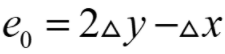斜率在0-1之间的Bresenham算法代码实现（替换上面程序中DDALine即可）：

void Bresenham_Line(int x0, int y0, int x1, int y1, HDC hdc)
{
int dx, dy, e, x=x0, y=y0;
dx = x1 - x0; dy = y1 - y0;
e = 2 * dy - dx;
while (x<=x1)
{
SetPixel(hdc, x, ScreenHeight-40-y, RGB(0, 0, 0));
if (e >= 0)//选右上方像素
{
e = e + 2 * dy - 2 * dx;
y++;
}
else//选右方像素
{
e = e + 2 * dy;
}
x++;
}
}

要实现任意方向的Bresenham算法也很容易，斜率在0-1之间意味着直线位于坐标系八象限中的第一象限，如果要绘制第二象限的直线，只需要利用这两个象限关于直线x=y对称的性质即可，可以先将x和y值互换先在第一象限进行计算，然后调用SetPixel时再将x和y值反过来，在第二象限中绘制，其他象限也是类似的思路。

绘制任意方向直线的Bresenham算法代码实现：

void Bresenham_Line(int x0, int y0, int x1, int y1, HDC hdc)
{
int flag = 0;
int dx = abs(x1 - x0);
int dy = abs(y1 - y0);
if (dx == 0 && dy == 0)   return;
if (abs(x1 - x0) < abs(y1 - y0))
{
flag = 1;
swap(x0, y0);
swap(x1, y1);
swap(dx, dy);
}
int tx = (x1 - x0) > 0 ? 1 : -1;
int ty = (y1 - y0) > 0 ? 1 : -1;
int x = x0;
int y = y0;
int dS = 2 * dy;   int dT = 2 * (dy - dx);
int e = dS - dx;
SetPixel(hdc, x0, y0, RGB(0,0,0));
while (x != x1)
{
if (e < 0)
e += dS;
else
{
y += ty;  e += dT;
}
x += tx;
if (flag)
SetPixel(hdc, y, ScreenHeight - 40 - x, RGB(0, 0, 0));
else
SetPixel(hdc, x, ScreenHeight - 40 - y, RGB(0, 0, 0));
}
}

二、圆形算法

1、中点画圆法

首先是中点画圆法，考虑圆心在原点，半径为R的圆在第一象限内的八分之一圆弧，从点（0, R）到点（R/ , R/ ）顺时针方向确定这段圆弧。假定某点Pi(xi, yi)已经是该圆弧上最接近实际圆弧的点，那么Pi的下一个点只可能是正右方的P1或右下方的P2两者之一，如图所示：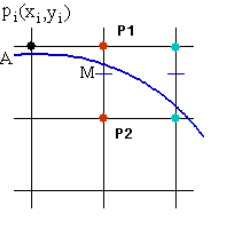构造判别函数：F(x, y）= x2 + y2 – R2

当F(x, y）= 0，表示点在圆上，当F(x, y）> 0，表示点在圆外，当F(x, y）< 0，表示点在圆内。如果M是P1和P2的中点，则M的坐标是（xi + 1, yi – 0.5），当F（xi + 1, yi – 0.5）< 0时，M点在圆内，说明P1点离实际圆弧更近，应该取P1作为圆的下一个点。同理分析，当F（xi + 1, yi – 0.5）> 0时，P2离实际圆弧更近，应取P2作为下一个点。当F（xi + 1, yi – 0.5）= 0时，P1和P2都可以作为圆的下一个点，算法约定取P2作为下一个点。

现在将M点坐标（xi + 1, yi – 0.5）带入判别函数F(x, y），得到判别式d：

d = F（xi + 1, yi – 0.5）= (xi + 1)2 + (yi – 0.5)2 – R2

若d < 0，则取P1为下一个点，此时P1的下一个点的判别式为：

d’ = F（xi + 2, yi – 0.5）= (xi + 2)2 + (yi – 0.5)2 – R2

展开后将d带入可得到判别式的递推关系：

d’ = d + 2xi + 3

若d > 0，则取P2为下一个点，此时P2的下一个点的判别式为：

d’ = F（xi + 2, yi – 1.5）= (xi + 2)2 + (yi – 1.5)2 – R2

展开后将d带入可得到判别式的递推关系：

d’ = d + 2(xi - yi) + 5

特别的，在第一个象限的第一个点（0, R）时，可以推倒出判别式d的初始值d0：

d0 = F(1, R – 0.5) = 1 – (R – 0.5)2 – R2 = 1.25 - R

根据上面的分析，可以写出中点画圆法的算法。考虑到圆心不在原点的情况，需要对计算出来的坐标进行了平移，下面就是通用的中点画圆法的源代码：

#include <stdio.h>
#include <graphics.h>
#include <conio.h>
#define x0 400
#define y0 300                    //定义全局变量x0,y0:坐标轴中心（x0,y0)
void Middle_point_draw_circle(int x1, int y1, int r)
{
int d0, x = 0, y = r;	//d0是判别式的值
d0 = 1.25 - r;   		//判别式的初始值，1.25可以改为1
while (x < y)
{
if (d0 >= 0)
{
d0 = d0 + 2 * (x - y) + 5;            //d0一定要先比x,y更新
x += 1;                				 //因为d0表达式中的x,y是上一个点
y -= 1;
putpixel(((x + x1) + x0), (y0 - (y + y1)), RED);         //(x,y)
putpixel(((-x + x1) + x0), (y0 - (y + y1)), RED);        //(-x,y)
putpixel(((y + x1) + x0), (y0 - (x + y1)), RED);         //(y,x)
putpixel(((-y + x1) + x0), (y0 - (x + y1)), RED);        //(-y,x)
putpixel(((x + x1) + x0), (y0 - (-y + y1)), RED);        //(x,-y)
putpixel(((-x + x1) + x0), (y0 - (-y + y1)), RED);       //(-x,-y)
putpixel(((y + x1) + x0), (y0 - (-x + y1)), RED);        //(y,-y)
putpixel(((-y + x1) + x0), (y0 - (-x + y1)), RED);       //(-y,-x)
Sleep(50);
}
else
{
d0 = d0 + 2 * x + 3;
x += 1;
y = y;
putpixel(((x + x1) + x0), (y0 - (y + y1)), RED);         //(x,y)
putpixel(((-x + x1) + x0), (y0 - (y + y1)), RED);        //(-x,y)
putpixel(((y + x1) + x0), (y0 - (x + y1)), RED);         //(y,x)
putpixel(((-y + x1) + x0), (y0 - (x + y1)), RED);        //(-y,x)
putpixel(((x + x1) + x0), (y0 - (-y + y1)), RED);        //(x,-y)
putpixel(((-x + x1) + x0), (y0 - (-y + y1)), RED);       //(-x,-y)
putpixel(((y + x1) + x0), (y0 - (-x + y1)), RED);        //(y,-y)
putpixel(((-y + x1) + x0), (y0 - (-x + y1)), RED);       //(-y,-x)
Sleep(50);
}
}
}
void main()
{
int x1, y1, r;
printf("请输入中点画圆算法圆心坐标(x1,y1)和圆的半径r:\n");
scanf("%d %d %d", &x1, &y1, &r);
initgraph(x0 * 2, y0 * 2);		    	//初始化图形窗口大小
setbkcolor(WHITE);
cleardevice();
setcolor(BLACK);
line(x0, 0, x0, y0 * 2);				//坐标轴X
line(0, y0, x0 * 2, y0);			 	//坐标轴Y
Middle_point_draw_circle(x1, y1, r);             //中点画圆算法
_getch();                                        //等待一个任意输入结束
closegraph();                                    //关闭图形窗口
}

2、Bresenham算法

中点画圆法中，计算判别式d使用了浮点运算，影响了圆的生成效率。如果能将判别式规约到整数运算，则可以简化计算，提高效率。于是人们针对中点画圆法进行了多种改进，其中一种方式是将d的初始值由1.25 – R改成1 – R，考虑到圆的半径R总是大于2，因此这个修改不会响d的初始值的符号，同时可以避免浮点运算。还有一种方法是将d的计算放大两倍，同时将初始值改成3 – 2R，这样避免了浮点运算，乘二运算也可以用移位快速代替，采用3 – 2R为初始值的改进算法，称为Bresenham算法。

源代码展示：

#include <stdio.h>
#include <graphics.h>
#include <conio.h>
#define x0 400
#define y0 300                    //定义全局变量x0,y0:坐标轴中心（x0,y0)
void Middle_point_draw_circle(int x1, int y1, int r)
{
int d0, x = 0, y = r;		//d0是判别式的值
d0 = 3 - 2*r;   			//判别式的初始值
while (x < y)
{
if (d0 >= 0)
{
d0 = d0 + 4 * (x - y) + 10;           	 //d0一定要先比x,y更新
x += 1;                					//因为d0表达式中的x,y是上一个点
y -= 1;
putpixel(((x + x1) + x0), (y0 - (y + y1)), RED);         //(x,y)
putpixel(((-x + x1) + x0), (y0 - (y + y1)), RED);        //(-x,y)
putpixel(((y + x1) + x0), (y0 - (x + y1)), RED);         //(y,x)
putpixel(((-y + x1) + x0), (y0 - (x + y1)), RED);        //(-y,x)
putpixel(((x + x1) + x0), (y0 - (-y + y1)), RED);        //(x,-y)
putpixel(((-x + x1) + x0), (y0 - (-y + y1)), RED);       //(-x,-y)
putpixel(((y + x1) + x0), (y0 - (-x + y1)), RED);        //(y,-y)
putpixel(((-y + x1) + x0), (y0 - (-x + y1)), RED);       //(-y,-x)
Sleep(50);
}
else
{
d0 = d0 + 4 * x + 6;
x += 1;
y = y;
putpixel(((x + x1) + x0), (y0 - (y + y1)), RED);         //(x,y)
putpixel(((-x + x1) + x0), (y0 - (y + y1)), RED);        //(-x,y)
putpixel(((y + x1) + x0), (y0 - (x + y1)), RED);         //(y,x)
putpixel(((-y + x1) + x0), (y0 - (x + y1)), RED);        //(-y,x)
putpixel(((x + x1) + x0), (y0 - (-y + y1)), RED);        //(x,-y)
putpixel(((-x + x1) + x0), (y0 - (-y + y1)), RED);       //(-x,-y)
putpixel(((y + x1) + x0), (y0 - (-x + y1)), RED);        //(y,-y)
putpixel(((-y + x1) + x0), (y0 - (-x + y1)), RED);       //(-y,-x)
Sleep(50);
}
}
}
void main()
{
int x1, y1, r;
printf("请输入中点画圆算法圆心坐标(x1,y1)和圆的半径r:\n");
scanf("%d %d %d", &x1, &y1, &r);
initgraph(x0 * 2, y0 * 2);		    	//初始化图形窗口大小
setbkcolor(WHITE);
cleardevice();
setcolor(BLACK);
line(x0, 0, x0, y0 * 2);				//坐标轴X
line(0, y0, x0 * 2, y0);			 	//坐标轴Y
Middle_point_draw_circle(x1, y1, r);             //中点画圆算法
_getch();                                        //等待一个任意输入结束
closegraph();                                    //关闭图形窗口
}

文章内容转自
https://www.cnblogs.com/LiveForGame/p/11706904.html
https://blog.csdn.net/l59565455/article/details/87473897

展开全文• DDA算法是计算机图形学中最简单的绘制直线算法。 已知直线段两端点P0(x0,y0)，P1(x1,y1)。 则可求得直线的斜率： k = (y2 - y1) / (x2 - x1) 在k，b均求出的条件下，只要知道一x值，我们就能计算出一y值。 ...

1、DDA（Digital Differential Analyzer）算法

DDA算法是计算机图形学中最简单的绘制直线算法。
已知直线段两个端点P0(x0,y0)，P1(x1,y1)
则可求得直线的斜率：

k = (y2 - y1) / (x2 - x1)

在k，b均求出的条件下，只要知道一个x值，我们就能计算出一个y值。

y = kx + b

如果横坐标x每次增加1（我们称其为步进为1，即x = x +1），那么y的步进就为k+b。

x = x + 1
y = y + (k + b)

同样知道一个y值也能计算出x值，此时y的步进为1，x的步进为(1-b)/k。

y = y + 1
x = x +(1 - b) / k

根据计算出的x值和y值，向下取整，得到坐标(x’,y’)，并在(x’,y’)处绘制直线段上的一点。

为进一步简化计算，通常可令b取0，将起点看作(0,0)。
设当前点的坐标为（xi ,yi),下一个像素点的坐标为（xi+1,yi+1)
则用DDA算法求解(xi+1，yi+1)的计算公式可以概括为：

xi+1 = xi + xStep (1)
yi+1 = yi + yStep (2)

我们一般通过计算 Δx 和 Δy 来确定xStep和yStep：

如果 Δx > Δy ，说明x轴的最大差值大于y轴的最大差值，x轴方向为步进的主方向，

xStep = 1，yStep = k；

如果 Δy> Δx，说明y轴的最大差值大于x轴的最大差值，y轴方向为步进的主方向，

yStep = 1，xStep = 1 / k。

根据这个公式，就能通过(xi，yi)迭代计算出(xi+1、yi+1)，然后在坐标系中绘制计算出的(x,y)坐标点。

实现工具：
1) VS2019(C++)

新建项目：2) 下载插件：Easyx 。使用方法和下载见官网： https://www.easyx.cn/
点击下载后安装在VC2019处点击安装源代码如下：

#include <iostream>
#include <graphics.h>
#include <math.h>
#include <conio.h>
using namespace std;

void DDALine(int x1, int y1, int x2, int y2)
{
int x0 = 400;
int y0 = 300;                  //记录原点坐标
int steps;                     //记录步长
int dx, dy;                    //记录起点和终点的坐标差值
float x, y;                    //记录即时坐标
float delta_x, delta_y;        //记录划线过程中的坐标增量
dx = x2 - x1;
dy = y2 - y1;
if (abs(dx) > abs(dy))         //比较横纵坐标增量的大小
steps = dx;
else
steps = dy;                //确保每次的增量不超过一个单位长度
x = x1;
y = y1;                        //记录画线起点
delta_x = float(dx*1.0) / abs(steps);
delta_y = float(dy*1.0) / abs(steps);   //计算相邻两个点的增量
putpixel(x, y, RED);
for (int i = 0; i <abs (steps); i++)
{
x = x + delta_x;
y = y + delta_y;
putpixel(x0 + int （x + 0.5, y0 - int(y + 0.5), RED);
Sleep(50);
}
}

int main()
{
int x1, x2, y1, y2;
int x0 = 400, y0 = 300;			                       //坐标轴中心（x0,y0)
cout << "请输入两个整数点的坐标(x1,y1),(x2,y2)" << endl;
cin >> x1 >> y1 >> x2 >> y2;
initgraph(x0 * 2, y0 * 2);		                       //初始化图形窗口大小
line(0, y0, x0 * 2, y0);			                   //坐标轴X
line(x0, 0, x0, y0 * 2);			                   //坐标轴Y
DDALine(x1, y1, x2, y2);                               //DDA画线算法
_getch();                                              //等待一个任意输入结束
closegraph();                                          //关闭图形窗口
return 0;
}

展开全文c++
• 显然，对于每一 xi的取值（x的变化规律是每次递增1），都有唯一的 yi值与之对应，通过对 yi值做舍五入运算，最终确定每一扫描列上的像素值。 但这种策略效率不高，因为每次迭代都要做一次浮点...DDA算法 计算机图形学 pyqt5 python
• 使用DDA算法画直线。 实验结果： 代码： 1 #include &lt;gl/glut.h&gt; 2 #include &lt;math.h&gt; 3 4 #define WIDTH 500 //窗口宽度 5 #define HEIGHT 500 //窗口高...
• 三种直线绘制方法：DDA算法、B算法和中点分割法。 在MFC环境中测试上述三种算法并对比分析三种算法的误差及效率。 二、程序框架 MFC程序： cgDrawLineView.h为视图层的头文件，负责声明各种成员变量和成员函数； ...c++ mfc
• DDA画直线算法

千次阅读 2010-02-12 22:26:00算法
• 计算机图形学DDA生成直线画法程序实验一、直线的生成一、实验目的掌握DDA直线画法、中点画线法和Bresenham画线法掌握VC++简单程序设计方法二、实验内容根据提供的程序框架，修改部分代码，完成画一条直线的功能(中点...
• DDA直线插补算法   数值微分法即DDA法(Digital Differential Analyzer)，是一种基于直线的微分方程来生成直线的方法。 直线DDA算法描述   设(x1，y1)和(x2，y2)分别为所求直线的起点和终点坐标，由直线的微分...dda算法 matlab
• 图形学初步---------直线生成算法DDA

万次阅读 多人点赞 2018-11-01 15:03:48
下面记录一下关于直线生成算法DDA的理解。 基础知识： 在计算机中，直线的显示并不是连续的，而是离散的点，这是由光栅化的本质决定的。 我们可以把屏幕理解为阴极射线管光栅显示器，这显示器是由离散可发光的...
• 1、调用画点函数，实现任意斜率直线绘制 （运用DDA算法、中点Bresenam算法实现并比较算法精度与效率） ② 基本论述 DDA算法又称数值微分法，是计算机图形学中一种基于直线的微分方程来生成直线的方法。 原理就是最...算法
• 计算机图形学直线生成算法实现.doc (7页) 本资源提供全文预览，点击全文预览即可全文预览,如果喜欢文档就下载吧，查找使用更方便哦！9.9 积分﻿实验二直线生成算法实现... 掌握几种基木的直线生成算法：DDA算法、br...
• 一、直线段的扫描转换算法 1.描述 数学上画一条直线需要无穷多的点，但计算机光栅显示器由像素点构成，故用屏幕上的点进行逼近。 为了在显示器上用离散的像素点逼近这条直线，首先需要知道这些像素点的（x,y）...算法
• 摘要：《计算机图形学》是计算机科学与技术专业一门重要的专业课，其中直线生成算法是教学重点之一。该文通过分析几种直线...DDA算法；Bresenham算法中图分类号：G642 文献标识码：A 文章编号：1009-3044(2015)23...
• 1、3单元 数字积分法插补原理,掌握数字积分法插补基本原理 掌握数字积分直线插补运算过程、特点及其应用 掌握数字积分圆弧插补运算过程、特点及其应用 理解改进数字积分插补质量的措施,本单元学习目标,一 基本原理,3...
• 直线生成的具体问题是已知直线的两端点,要求在图像输出设备上从起点到中点通过逐次循环迭代，找到最接近直线的像素点集合。 本次实验所涉及的算法有三种：DDA算法、B算法和重点分割法。在MFC环境中测试三种算法并...C++ MFC
• 该算法可以说是前两种的升级版，它不仅有一高效的算法效率，它的适用范围也更广，可以用来画曲线等等。该算法的思想是通过各行、各列像素中心构造一组虚拟网格线，按照直线起点到终点的顺序，计算直线与各垂直网格...
• 计算机图形学复习2

千次阅读 2020-08-18 22:10:37算法 直线生成算法 圆弧生成算法
• 此系列是对年专业课程学习的回顾，索引参见：http://www.voidcn.com/article/p-srsfcefa-vo.html直线DDA算法【算法介绍】设直线之起点为(x1，y1)，终点为(x2，y2)，则斜率m为： 直线中的每一点坐标都可以由前...
• MFC基本图形的绘制

千次阅读 2019-12-04 23:56:06
通过本次实验，掌握Behamsen或DDA方法绘制直线、圆或椭圆算法原理，加深对算法的理解，并采用Vc++提供的绘图函数实现多义线、矩形、多边形的绘制。了解区域填充的实现过程。 二、实验环境 1.硬件环境：PC机 2.软件...
• 直线绘制算法

2010-04-15 00:19:00
以前在学校里学过图形学，但没用过功，效果很不好。...算法一， // 直线DDA(数值微分)算法// 直线起始点(xa, ya)// 直线终止点(xa, ya)void lineDDA(int xa, int ya, int xb, int yb, HDC hDC, COLORREF cr算法 float 图形
• 直线生成算法

千次阅读 2012-03-26 20:48:07
直线生成算法：在光栅系统中绘制直线段，需要采用直线生成算法，常用的有DDA算法、Bresenham算法等。 Ø DDA直线生成算法是一种使用微分方程生成直线的算法，又称作数值微分法。该算法的基本思想是：根据斜率确定...算法 mfc float
• 注：比如业务层一动画、一复杂的图形，对于画直线函数的调用可能是成百上千次，甚至是成千上万次的，所以图元算法的优劣对性能的影响是非常大的。 二、什么是OpenGL OpenGL的英文名称：Open Graph...
• 直线的光栅化算法 write by 九天雁翎(JTianLing) -- blog.csdn.net/vagrxie 讨论新闻组及文件 看"参考1"碰到的第一算法，有点意思，总结一下，特别是因为"参考1"...
• 此系列是对年专业课程学习的回顾，索引参见：http://blog.csdn.net/xiaowei_cqu/article/details/7747205直线DDA算法【算法介绍】设直线之起点为(x1，y1)，终点为(x2，y2)，则斜率m为：直线中的每一点坐标都可以...
• 消隐的分类，如何消除隐藏线、隐藏面，主要介绍以下几算法（他们都属于图像空间的消隐算法）： Z缓冲区(Z-Buffer)算法扫描线Z-buffer算法区域子分割算法 1、消隐  当我们观察空间任何一不透明的物体时...